# Image scale

The actual image dimensions are 60 cm x 80cm and has a reduced size 3 cm x 4 cm. At what scale the image was reduced?

M 1:  20

### Step-by-step explanation: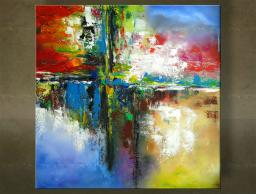Did you find an error or inaccuracy? Feel free to write us. Thank you!Tips to related online calculators
Check out our ratio calculator.

## Related math problems and questions:

• A mapA map of size 60cm by 25cm is reduced in the ratio of 3:5, twice. What are the new dimensions?
• Plan scale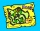The actual width of the room is 66 dm, the width in plan is 4.4 cm. What scale corresponds to the plan?
• The scaleThe distance of 15 cm on the map corresponds to a distance of 27 km in reality. What is the scale of the map?
• Fence and scaleThe garden with 80 m of fencing is draw on plan as a square with a side length of 4 cm. At what scale is this plan made?
• Map 2At what scale is made map if the distance 8.2 km corresponds on the map segment 5 cm long?
• Scale of the mapDetermine the scale of the map if the actual distance between A and B is 720 km and the distance on the map is 20 cm.
• Scale of the mapDetermine the map's scale, which is the actual distance of 120 km l represented by a segment long 6 cm.
• On the mapA line 1.5 cm long corresponds to a line 3 cm long on the map. What is the scale of the map?
• Map scaleThe rectangular plot has a scale of 1: 10000 area 3 cm2 on the map. What content does this plot have on a 1:5000 scale map?
• Map scale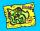What is the scale of the map, if 5.4 km is 72 mm on the map?
• A map 4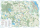A map represents every 4 miles with 1 inch. If a school and a bank are actually 12 miles apart, how far apart are they on the map, in inches?
• Scale 3Miriam room is 3.2 meters wide. It is draw by line segment length 6.4 cm on floor plan. In what scale it is plan of the room?
• Hectares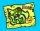Determine the area of the rectangular land (in ha), which has dimensions of 3 cm and 4.5 cm on the plan with a scale of 1: 40,000.
• Scale of the map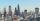The distance between two cities is actually 30 km and the map is 6 cm. What is the scale of the map?
• A rectangle 8A rectangle measuring 6 cm and 4 cm is enlarged by the ratio 3:1. What is the area of the enlarged rectangle?
• Map scaleGarden has on plan on a scale of 1: 150 width 22 cm and length 35 cm. What is the real area of the garden?
• Two discounts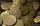The MP4 player cost 960 CZK, the price was twice gradually reduced in the ratio 4: 3: 3/2. How much did the player cost after the second discount?